10.1 Non-right triangles: law of sines  (Page 5/10)

 Page 5 / 10

Verbal

Describe the altitude of a triangle.

The altitude extends from any vertex to the opposite side or to the line containing the opposite side at a 90° angle.

Compare right triangles and oblique triangles.

When can you use the Law of Sines to find a missing angle?

When the known values are the side opposite the missing angle and another side and its opposite angle.

In the Law of Sines, what is the relationship between the angle in the numerator and the side in the denominator?

What type of triangle results in an ambiguous case?

A triangle with two given sides and a non-included angle.

Algebraic

For the following exercises, assume $\text{\hspace{0.17em}}\alpha \text{\hspace{0.17em}}$ is opposite side $\text{\hspace{0.17em}}a,\beta \text{\hspace{0.17em}}$ is opposite side $\text{\hspace{0.17em}}b,\text{\hspace{0.17em}}$ and $\text{\hspace{0.17em}}\gamma \text{\hspace{0.17em}}$ is opposite side $\text{\hspace{0.17em}}c.\text{\hspace{0.17em}}$ Solve each triangle, if possible. Round each answer to the nearest tenth.

$\alpha =43°,\gamma =69°,a=20$

$\alpha =35°,\gamma =73°,c=20$

$\alpha =60°,\text{\hspace{0.17em}}\text{\hspace{0.17em}}\beta =60°,\text{\hspace{0.17em}}\gamma =60°$

$a=4,\text{\hspace{0.17em}}\text{\hspace{0.17em}}\alpha =\text{\hspace{0.17em}}60°,\text{\hspace{0.17em}}\beta =100°$

$b=10,\text{\hspace{0.17em}}\beta =95°,\gamma =\text{\hspace{0.17em}}30°$

For the following exercises, use the Law of Sines to solve for the missing side for each oblique triangle. Round each answer to the nearest hundredth. Assume that angle $\text{\hspace{0.17em}}A\text{\hspace{0.17em}}$ is opposite side $\text{\hspace{0.17em}}a,\text{\hspace{0.17em}}$ angle $\text{\hspace{0.17em}}B\text{\hspace{0.17em}}$ is opposite side $\text{\hspace{0.17em}}b,\text{\hspace{0.17em}}$ and angle $\text{\hspace{0.17em}}C\text{\hspace{0.17em}}$ is opposite side $\text{\hspace{0.17em}}c.$

Find side $\text{\hspace{0.17em}}b\text{\hspace{0.17em}}$ when $\text{\hspace{0.17em}}A=37°,\text{\hspace{0.17em}}\text{\hspace{0.17em}}B=49°,\text{\hspace{0.17em}}c=5.$

$b\approx 3.78$

Find side $\text{\hspace{0.17em}}a$ when $\text{\hspace{0.17em}}A=132°,C=23°,b=10.$

Find side $\text{\hspace{0.17em}}c\text{\hspace{0.17em}}$ when $\text{\hspace{0.17em}}B=37°,C=21,\text{\hspace{0.17em}}b=23.$

$c\approx 13.70$

For the following exercises, assume $\text{\hspace{0.17em}}\alpha \text{\hspace{0.17em}}$ is opposite side $\text{\hspace{0.17em}}a,\beta \text{\hspace{0.17em}}$ is opposite side $\text{\hspace{0.17em}}b,\text{\hspace{0.17em}}$ and $\text{\hspace{0.17em}}\gamma \text{\hspace{0.17em}}$ is opposite side $\text{\hspace{0.17em}}c.\text{\hspace{0.17em}}$ Determine whether there is no triangle, one triangle, or two triangles. Then solve each triangle, if possible. Round each answer to the nearest tenth.

$\alpha =119°,a=14,b=26$

$\gamma =113°,b=10,c=32$

one triangle, $\text{\hspace{0.17em}}\alpha \approx 50.3°,\beta \approx 16.7°,a\approx 26.7$

$b=3.5,\text{\hspace{0.17em}}\text{\hspace{0.17em}}c=5.3,\text{\hspace{0.17em}}\text{\hspace{0.17em}}\gamma =\text{\hspace{0.17em}}80°$

$a=12,\text{\hspace{0.17em}}\text{\hspace{0.17em}}c=17,\text{\hspace{0.17em}}\text{\hspace{0.17em}}\alpha =\text{\hspace{0.17em}}35°$

two triangles, or

$a=20.5,\text{\hspace{0.17em}}\text{\hspace{0.17em}}b=35.0,\text{\hspace{0.17em}}\text{\hspace{0.17em}}\beta =25°$

$a=7,\text{\hspace{0.17em}}c=9,\text{\hspace{0.17em}}\text{\hspace{0.17em}}\alpha =\text{\hspace{0.17em}}43°$

two triangles, or

$a=7,b=3,\beta =24°$

$b=13,c=5,\gamma =\text{\hspace{0.17em}}10°$

two triangles, $\text{\hspace{0.17em}}\alpha \approx 143.2°,\beta \approx 26.8°,a\approx 17.3\text{\hspace{0.17em}}$ or $\text{\hspace{0.17em}}{\alpha }^{\prime }\approx 16.8°,{\beta }^{\prime }\approx 153.2°,{a}^{\prime }\approx 8.3$

$a=2.3,c=1.8,\gamma =28°$

$\beta =119°,b=8.2,a=11.3$

no triangle possible

For the following exercises, use the Law of Sines to solve, if possible, the missing side or angle for each triangle or triangles in the ambiguous case. Round each answer to the nearest tenth.

Find angle $A$ when $\text{\hspace{0.17em}}a=24,b=5,B=22°.$

Find angle $A$ when $\text{\hspace{0.17em}}a=13,b=6,B=20°.$

$A\approx 47.8°\text{\hspace{0.17em}}$ or $\text{\hspace{0.17em}}{A}^{\prime }\approx 132.2°$

Find angle $\text{\hspace{0.17em}}B\text{\hspace{0.17em}}$ when $\text{\hspace{0.17em}}A=12°,a=2,b=9.$

For the following exercises, find the area of the triangle with the given measurements. Round each answer to the nearest tenth.

$a=5,c=6,\beta =\text{\hspace{0.17em}}35°$

$8.6$

$b=11,c=8,\alpha =28°$

$a=32,b=24,\gamma =75°$

$370.9$

$a=7.2,b=4.5,\gamma =43°$

Graphical

For the following exercises, find the length of side $\text{\hspace{0.17em}}x.\text{\hspace{0.17em}}$ Round to the nearest tenth.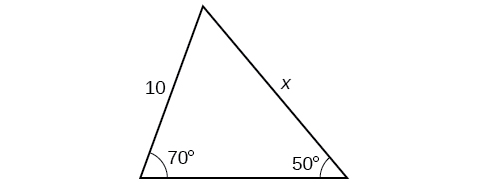$12.3$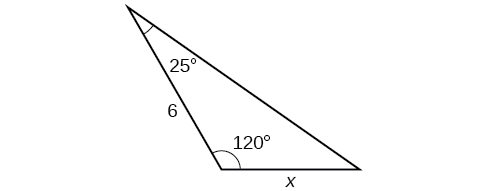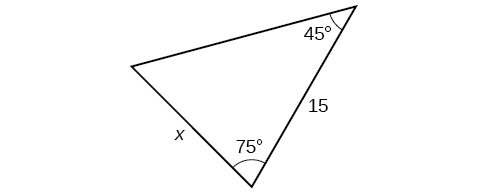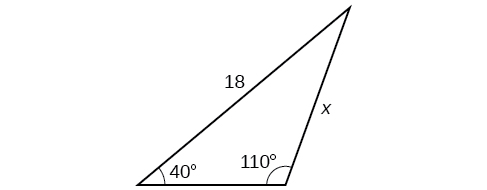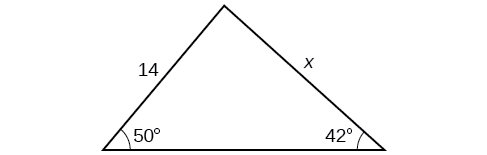For the following exercises, find the measure of angle $\text{\hspace{0.17em}}x,\text{\hspace{0.17em}}$ if possible. Round to the nearest tenth.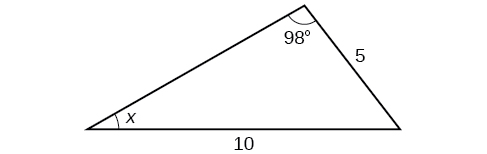$29.7°$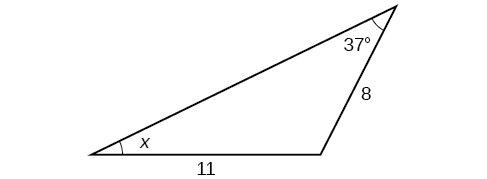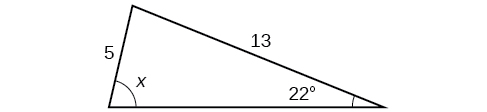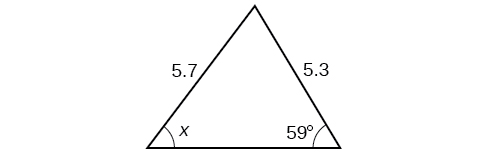Notice that $\text{\hspace{0.17em}}x\text{\hspace{0.17em}}$ is an obtuse angle.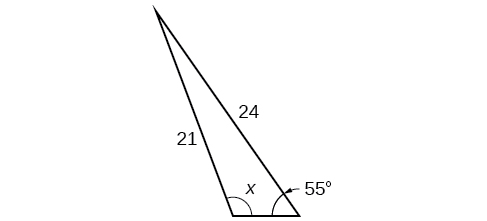$110.6°$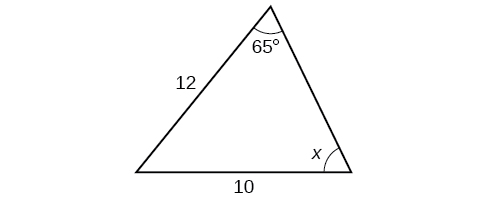For the following exercises, find the area of each triangle. Round each answer to the nearest tenth.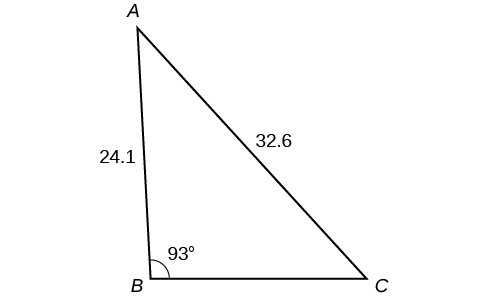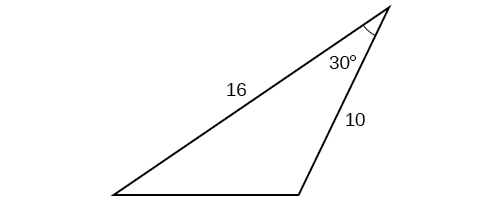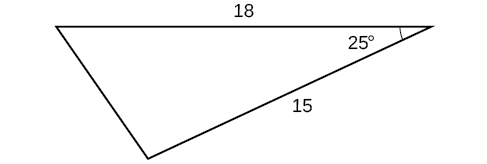$57.1$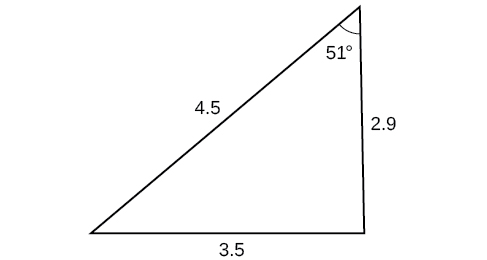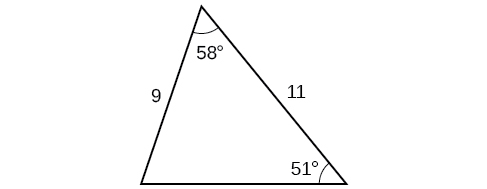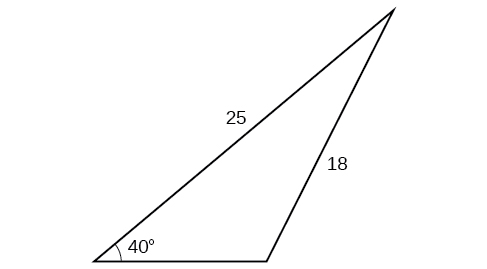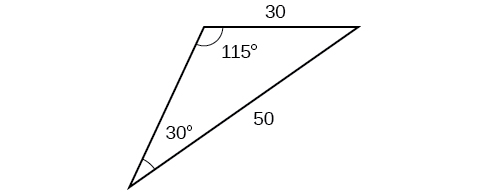Extensions

Find the radius of the circle in [link] . Round to the nearest tenth.

Find the diameter of the circle in [link] . Round to the nearest tenth.

$10.1$

The sequence is {1,-1,1-1.....} has
how can we solve this problem
Sin(A+B) = sinBcosA+cosBsinA
Prove it
Eseka
Eseka
hi
Joel
June needs 45 gallons of punch. 2 different coolers. Bigger cooler is 5 times as large as smaller cooler. How many gallons in each cooler?
7.5 and 37.5
Nando
find the sum of 28th term of the AP 3+10+17+---------
I think you should say "28 terms" instead of "28th term"
Vedant
the 28th term is 175
Nando
192
Kenneth
if sequence sn is a such that sn>0 for all n and lim sn=0than prove that lim (s1 s2............ sn) ke hole power n =n
write down the polynomial function with root 1/3,2,-3 with solution
if A and B are subspaces of V prove that (A+B)/B=A/(A-B)
write down the value of each of the following in surd form a)cos(-65°) b)sin(-180°)c)tan(225°)d)tan(135°)
Prove that (sinA/1-cosA - 1-cosA/sinA) (cosA/1-sinA - 1-sinA/cosA) = 4
what is the answer to dividing negative index
In a triangle ABC prove that. (b+c)cosA+(c+a)cosB+(a+b)cisC=a+b+c.
give me the waec 2019 questions
the polar co-ordinate of the point (-1, -1)ByByByBy